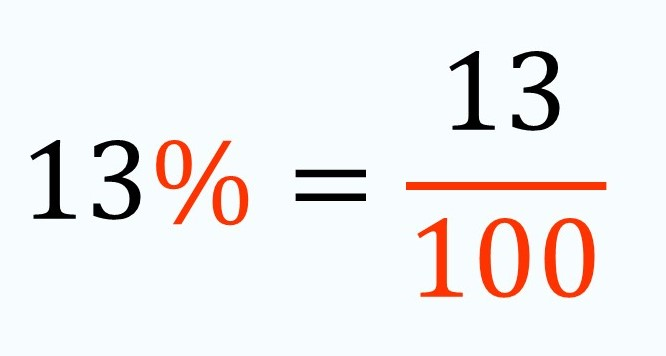Smartick is a fun way to learn math!Oct15

# Percentage: What Is It and How do We Calculate It?

If we talk about percentages, it seems to be a familiar topic but: Would we know what a percentage is? How it is calculated? And what it means exactly? This post is going to resolve all these doubts, let’s get to it!

#### A percentage is, actually, a symbol.

A symbol that represents a fraction with 100 as its denominator. So, in written language, it is much easier to write the percentage rather than the fraction:We read this symbol (%) as ”percent” and it indicates, like we had said before, the number of parts of a unit, or a reference amount, that has been divided. Therefore, percentage (%) always appears in an expression that relates two quantities. For example, ”13% of children that do Smartick chose to play the game Entangled after their session.” In this case, the quantities that are being related are the number of children that do Smartick and the amount of those children that chose to play Entangled after their session.But, how could we calculate the exact number of children that chose to play Entangled? Well, it´s very simple, but we should know a few things:

• How to multiply fractions.
• How many children do Smartick: imagine that there are only 300 children.Since we know the reference amount (the number of children that do Smartick) we only have to multiply the fraction to indicate the percentage, 13%, to say ”13 parts of 100” for 300. What we want to do is divide the reference amount into 100 equal parts and then take 13.

So, 300 divided by 100 is 3. Which multiplied by 13 is 39.  Therefore, 39 of 300 Smartick students chose the game Entangled after their sessions.

Now, if you were interested in this post and would like to continue learning, go to Smartick and try it for free.